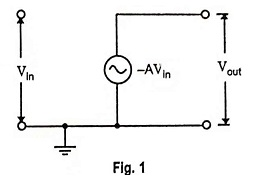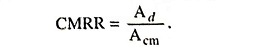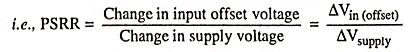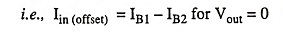## Operational Amplifiers Interview Questions and Answers:

1. What is an op-amp?

Ans. The name operational amplifier stems from its original use for mathematical operations (such as addition, subtraction, multiplication, differentiation and integration) and is abbreviated as op-amp. It is a direct-coupled high gain amplifier usually consisting of one or more differential amplifiers and usually followed by a level translator and an output stage (usually a push-pull or push-pull complementary-symmetry pair). With the addition of suitable external feedback components, the modern day op-amp can be employed for a variety of applications.

2. Write the list of applications of op-amp.

Ans. An op-amp is so named as it was originally designed to perform mathematical operations such as summation, subtraction, multi­plication, differentiation and integration etc. Nowadays, the op-amps are put to a variety of other uses such as sign changing, scale changing, phase shifting, voltage regulation, analog computer operations, in instrumentation and control systems, oscilla­tor circuits, pulse generators, square-wave generators, triangular-wave generators, comparators, analog-to-digital and digital-to­-analog converters (ADC and DAC); voltage-to-current convert­ers, current-to-voltage converters, sample and hold circuits etc.

3. Explain why the input terminals of an op-amp are named as inverting input and noninverting input?

Ans. Op-amp has two input terminals namely inverting and noninverting input terminals.

The input terminal marked with plus (+) is named as noninverting input terminal because a signal applied to this input terminal appears with the same polarity and amplified at the output. On the other hand, the input terminal marked with minus (-) is named as inverting input terminal because a sig­nal applied to this terminal appears amplified but inverted at the output.

4. What is the maximum value of output voltage obtainable from an op-amp?

Ans. The output voltage magnitude is limited by supply voltages. The maximum output voltage will be about 2 or 3 volts less than the supply voltages VCC.

5. What are the characteristics of ideal op-amp?

Ans. Assumptions made for analyzing ideal op-amp are (i) infinite open-loop gain (ii) infinite input impedance (iii) zero output impedance (iv) perfect balance (v) infinite frequency bandwidth, (vi) infinite slew rate (vii) infinite common-mode rejection ratio and (viii) nil drift of characteristics with temperature.

6. Write the input and output resistance of an ideal operational amplifier.

Ans. Input resistance of an ideal op-amp is ∞ (infinity).

Output resistance of an ideal op-amp is 0 (zero).

7. Draw the equivalent circuit of an ideal op-amp.

Ans. Equivalent circuit of an ideal op-amp is shown in Fig. 1.8. What is a voltage transfer curve of an op-amp?

Ans. The curve drawn between output voltage and input differen­tial voltage, for an op-amp, keeping voltage gain A constant is known as voltage transfer curve.

9. What are differential gain and common-mode gain of a differential amplifier?

Ans. When the difference of the two inputs applied to the two terminals of a differential amplifier is amplified, the resultant gain is termed as differential gain. But when the two input terminals are connected to the same input source then the gain established by the differential amplifier is called the common mode gain.

10. Define CMRR. Explain with example.

Ans. CMRR is defined as the ratio of differential voltage gain to common-mode voltage gain and it is given asHigh CMRR ensures that the common mode signals such as noise are rejected successfully and the output voltage is proportional only to the differential input voltage.

11. Why does an op-amp have high CMRR?

Ans. High CMRR ensures that the common mode signals such as noise are rejected successfully and the output voltage is proportional only to the differential input voltage.

12. What are the applications of op-amp in open-loop configuration?

Ans. Applications of op-amp in open-loop configuration are:

1. Differential amplifier, 2. Inverting amplifier, 3. Noninverting amplifier.

13. Why open-loop op-amp configuration is unsuitable for linear applications?

Ans. When an op-amp is operated in the open-loop configuration, the output either goes to positive saturation or negative saturation levels or switches between positive and negative saturation levels and thus clips the output above these levels. So open-loop op-amp configurations are not used in linear applications.

14. What is offset voltage?

Ans. Input offset voltage may be defined as that voltage which is to be applied between the input terminals to balance the amplifier.

15. Give the typical value of bias current for CA741 operational amplifier.

Ans. 80nA.

16. If the currents flowing towards the inverting and noninverting terminals of an op-amp are 3 μA and 1 μA respectively, what is the input bias current and the input offset current?

Ans. 2 µA and 2 µA.

17. What is slew rate? List the causes of slew rate and explain its significance in applications.

Ans. Slew rate of an op-amp is defined as the maximum rate of change of output voltage per unit time and is expressed in V/μs.

Slew rate is caused by current limiting and saturation of internal stages of an op-amp when a high frequency large amplitude signal is applied.

Slew rate decides capability of op-amp to change its output rapidly hence it decides high frequency of operation. It changes with change in voltage gain.

The slew rate has important effects on both open-loop and closed-loop op-amp circuits. The slew-rate limitations can also affect the performance of the circuit when handling large sinusoidal signals at higher frequencies.

18. What is slew rate?

Ans. Slew rate of an op-amp is defined as the maximum rate of change of output voltage per unit time and is expressed in V/μs.

19. Define PSRR.

Ans. The power supply rejection ratio (PSRR) is defined as the ratio of the change in input offset voltage to the corresponding change in one power supply voltage, with all remaining power supply voltages kept constant.It is also called the supply voltage rejection ratio (SVRR) or power supply sensitivity (PSS). It is expressed in μV per volt or in decibels. Lower value of PSRR in μV/V is an indication of better performance of the op-amp. It ranges from 10-5 to 7 x 10-5.

20. What is input offset current?

Ans. Input offset current Iin(offset) is defined as the difference between the two currents entering the input terminals of a balanced amplifier for Vout = 021. Name three important specifications of IC op-amps.

Ans. The three important specifications of IC op-amps are high voltage gain, low output impedance and high input impedance.

22. What is voltage gain of a practical op-amp?

Ans. Voltage gain of a practical op-amp is of the order of 105.

Scroll to Top